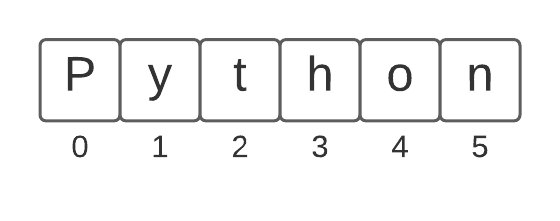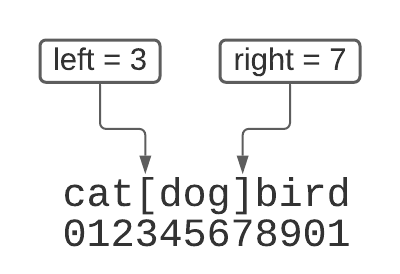Computer Science

# More strings

## Concepts from last time

• strings indexed starting from zero
• get a character with square brackets `s` or `s[i]`
• `len(s)` — length of a string
• various string functions like `s.isalpha()`### `str_dx(s)` and the accumulator pattern

``````def str_dx(s):
result = ''
for i in range(len(s)):
if s[i].isdigit():
result += 'd'
else:
result += 'x'
return result

str_dx("I'm 91. You call me old??! I'm wise and experienced!")``````
``    'xxxxddxxxxxxxxxxxxxxxxxxxxxxxxxxxxxxxxxxxxxxxxxxxxxx'``

### `count_e(s)` problem and the accumulator pattern

• count the number of ‘e’ characters in a string
• accumulator pattern using an integer variable
``````def count_e(s):
count = 0
for i in range(len(s)):
if s[i] == 'e':
# count = count + 1
count += 1
return count

result = count_e('abcdefg')
print(result)
result = count_e('abcdefgeabce')
print(result)``````
``````    1
3``````

### `has_alpha(s)` function, early return pattern, doctests

``````def has_alpha(s):
"""
Returns true if there are any alphabetic characters in the string, false otherwise.

:param s: a string
:return: True if there are alphabetic characters in s, otherwise False
>>> has_alpha('45#a8e')
True
>>> has_alpha('45^#)-')
False
"""
for i in range(len(s)):
if s[i].isalpha():
return True
return False``````

### if statements

``````if something_is_true:
do_things()
else:
do_other things()

if not something_is_true:
do_things()

if first_check:
do_first_things()
elif second_check:
do_second_things()
elif third_check:
do_third_things()
else:
do_fourth_things()``````

## `in` test

• check for presence of a string in another string
• also a `not in` variant
``````'Dog' in 'CatDogBird'

'dog' in 'CatDogBird'   # upper vs. lower case

'd' in 'CatDogBird'     # finds d at the end

'i' in 'CatDogBird'     # finds lower case characters

'x' not in 'CatDogBird' # also have a "not in" variant

s = 'my birthday party'
if 'birth' in s:
print('birth is in this string')``````

### `has_pi(s)` function

``````def has_pi(s):
""" return true if "3" and "14" are in the string (not necessarily next to each other)
"""
if '3' in s and '14' in s:
return True
return False

print(has_pi('3.1415'))
print(has_pi('Today is the 3rd time in 14 days that I have slept until 10am.'))
print(has_pi('Which 3 players are your favorite?'))``````
``````    True
True
False``````

### `find_cat(s)` function

• look for instances of ‘c’, ‘a’, ‘t’, ‘C’, ‘A’, ‘T’
• return a new string that has just these characters, in the order they appear
sreturn value
xCtxxxAaxCtAa
xaCxxxTxaCT

### `find_cat(s)` attempt #1

• misses the capital letters
``````def find_cat(s):
"""
>>> find_cat('xCtxxxAax')
'CtAa'
>>> find_cat('xaCxxxTx')
aCT
"""
result = ''
for i in range(len(s)):
if s[i] == 'c' or s[i] == 'a' or s[i] == 't':
result += s[i]
return result

print(find_cat('xCtxxxAax'))
print(find_cat('xaCxxxTx'))``````
``````    ta
a``````

### `find_cat(s)` attempt #2

• do 6 comparisons!
• works but it is ugly

### `find_cat(s)` attempt #3

• convert character to lowercase first (doesn’t modify the original string)
• makes it easier to test without having to write 6 comparisons
• still a long comparison
``````def find_cat(s):
result = ''
for i in range(len(s)):
if s[i].lower() == 'c' or s[i].lower() == 'a' or s[i].lower() == 't':
result += s[i]
return result

print(find_cat('xCtxxxAax'))
print(find_cat('xaCxxxTx'))``````

### `find_cat(s)` attempt #3

• decompose with a varible
• compute `s[i].lower()` once, store it in a variable
• avoids repetition
• code is easier to read
• decomposition within a function — do small pieces at a time
``````def find_cat(s):
result = ''
for i in range(len(s)):
low = s[i].lower()   # decompose with a variable
if low == 'c' or low == 'a' or low == 't':
result += s[i]
return result

print(find_cat('xCtxxxAax'))
print(find_cat('xaCxxxTx'))``````
``````    CtAa
aCT``````

### a note on variable names

• don’t use ‘lower’ — duplicates a function name
• could use ‘lower_case_char’ — something descriptive
• use underscores between words

### `find_cat(s)` attempt #4

• can make this a little bit simpler
• instead of three comparisons, use `low in 'cat'`
``````def find_cat(text):
result = ''
for index in range(len(text)):
low = text[index].lower()
if low == 'c' or low == 'a' or low == 't':
if low in 'cat':
result += text[index]
return result

print(find_cat('xCtxxxAax'))
print(find_cat('xaCxxxTx'))``````
``````    CtAa
aCT``````

## `s.find(target)`

• find a `target` string inside of `s`
• returns the index where it is found
``````s = 'Python'
print('th: ',s.find('th'))
print('o: ',s.find('o'))
print('y: ',s.find('y'))
print('x: ', s.find('x'))
print('N: ',s.find('N'))
print('P: ',s.find('P'))``````
``````    th:  2
o:  4
y:  1
x:  -1
N:  -1
P:  0``````

## String slicing

• get a substring from a string
• used very heavily
• use brackets and then `start_index:end_index`
• returned string is from `start_index` to `end_index-1`
``````s = 'cats and dogs'

s[0:4]
'cats'

s[2:6]
'ts a'

s[9:13]
'dogs'``````

### can leave off either the start or the end indexing

• if you leave off the start, it starts at the start (0)
• if you leave off the end, it ends at the end (`len(s)`)
``````s = 'cats and dogs'

s[0:4]
s[:4]
'cats'

s[9:13]
s[9:]
'dogs'``````

### edge cases

``````
s = 'cats and dogs'

s[3:3]   # starting and ending the same -> empty string
''

s[9:999] # ending too big -> end
'dogs'

s[:]     # the whole string
'cats and dogs'

s[5:-2]  # negative numbers at the end counts backward from the end
'and do'
''``````

## `brackets(s)` problem

• look for a pair of brackets ’[…]’ within s
• return the text between the brackets
• if there are no brackets, return the empty string
• assume if there is a left bracket, there will always be a right bracket after it
• assume if brackets are present, there will be only one of each
sreturn value
‘cat[dog]bird’‘dog’
‘catdogbird’

### draw it out!

• find the index of the left bracket and the right bracket### `brackets(s)` solution

``````def brackets(s):
left = s.find('[')
if left == -1:
return ''
right = s.find(']')
if right == -1:
right = len(s)
return s[left+1:right]

result = brackets('cat[dog]bird')
print(result)
result = brackets('catdogbird')
# this prints the empty string
print(f"[{result}]")
result = brackets('cat[dogbird')
print(result)``````
``````    dog
[]
dogbird``````

## `s.find(target, start_index)`

• find `target` inside the string, but starting at `start_index`
``````s = '[xyz['

s.find('[')      # find first [
0

s.find('[', 1)   # start search at 1
4``````

### `parens(s)` problem

• like brackets, but use parentheses
• may have other parentheses mixed in
• write this in PyCharm, with doctests
• can you help write the doctests?
sreturn value
‘x)x(abc)xxx’‘abc’

### `parens(s)` solution

``````def parens(s):
"""
Return the substring that is inside the first set of matching parentheses

:param s: a string
:return: a substring that is inside the first set of matching parentheses

>>> parens('x)x(abc)xxx')
'abc'
"""
left = s.find('(')
right = s.find(')', left + 1)
return s[left+1:right]``````